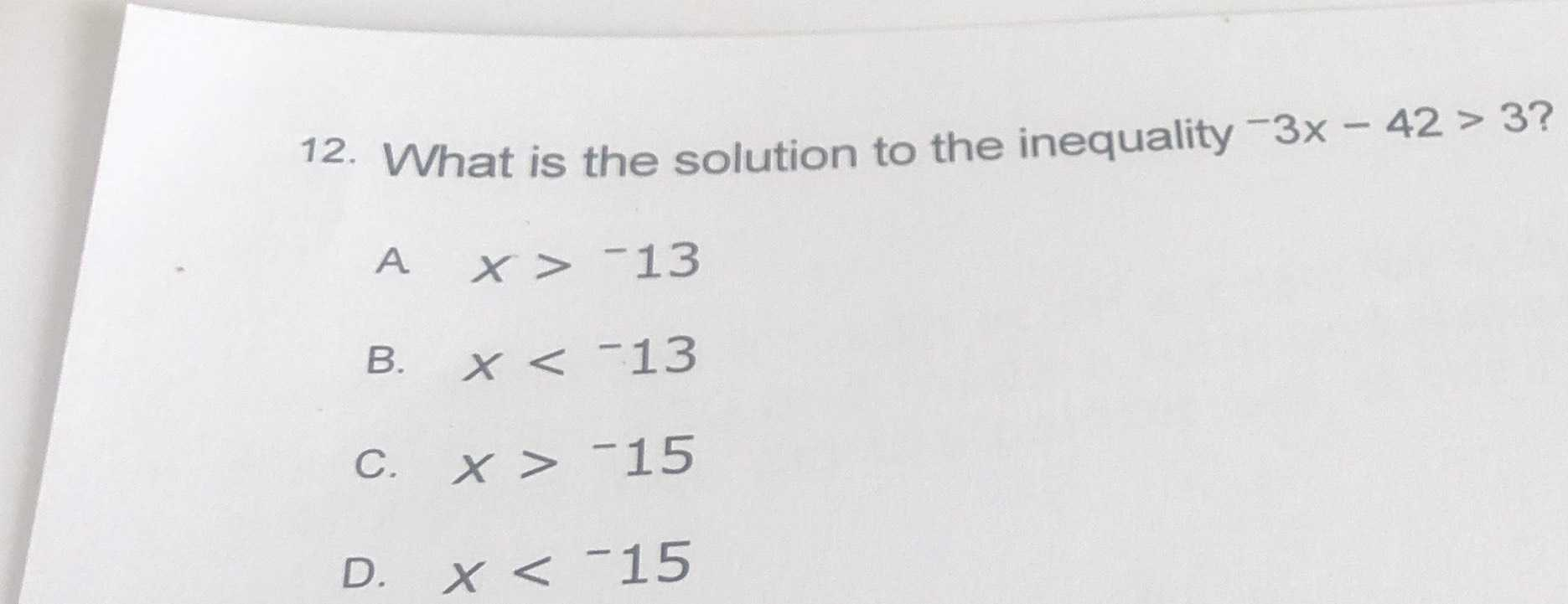### ¿Todavía tienes preguntas de matemáticas?

Pregunte a nuestros tutores expertos
Algebra
Pregunta12. What is the solution to the inequality $$- 3 x - 42 > 3$$ ? A $$x > - 13$$

B. $$x < - 13$$

C. $$x > - 15$$

D. $$x < - 15$$